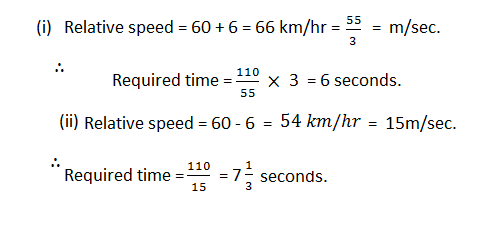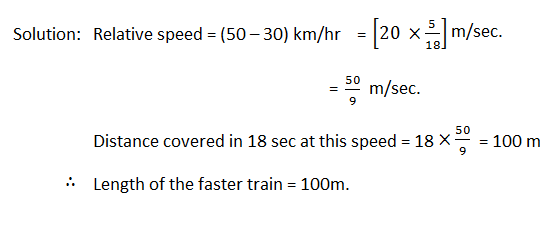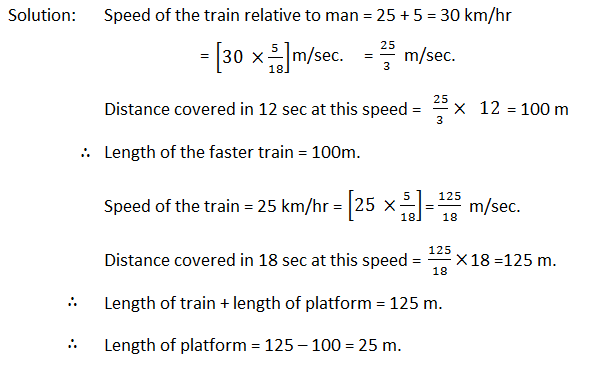# Problems on Trains - Types of Problems with Examples

Problems on Trains chapter is almost same as TIME AND DISTANCE. The only difference is that the length of the moving object is also considered.### Some important things to be noticed:

(i) When two trains are moving in opposite directions, their speeds should be added to find the relative speed.

(ii) When they are moving in the same direction, the relative speed is the difference of their speeds.

(iii) When a train passes a platform, it should travel the length equal to the sum of the lengths of trains & platform both.

### Trains passing a telegraph post or a stationary man

1.How many seconds will a train 100 metres long running at the rate of 36 km an hour take to pass a certain telegraph post?

Solution: In passing the post the train must travel its own length.
Now,    36 km/hr     =     36 ×5/18     =     10 m/sc.
Required time = 100/10 = 10 seconds.

### Trains crossing a bridge or passing a railway station

2.How long does a train 110 metres long running at the rate of 36 km/hr take to cross a bridge 132 metres in length?### Trains running in opposite direction

3.Two trains 121 metres and 99 metres in length respectively are running in opposite directions, one at the rate of 40 km and the other at the rate of 32 km an hour. In what time will they be completely clear of each other from the moment they meet?### Trains running in the same direction

4. In example above. If the trains were running in the same
direction, in what time will they be clear each other?### Trains passing a man who is walking

5. A train 110 metres in length travels at 60 km/hr. In what time will it pass a man who is walking at 6 km an hour (i) against it; (ii) in the same direction?

Solution: This question is to be solved like the above examples 3 and 4, the  only difference being that the length of the man is zero.6. Two trains are moving in the same direction at 50 km/hr and 30 km/hr. The faster train crosses a man in the slower train in 18 seconds. Find the length of the faster train.7. A train running at 25 km/hr takes 18 seconds to pass a platform. Next, it takes 12 seconds to pass a man walking at 5 km/hr in the opposite direction. Find the length of the train and that of the platform.#### What's trending in BankExamsToday

Smart Prep Kit for Banking Exams by Ramandeep Singh - Download here